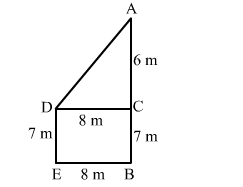# Two poles of height 13 m and 7 m respectively stand vertically on a plane ground at a distance of 8 m from each other.Question:

Two poles of height 13 m and 7 m respectively stand vertically on a plane ground at a distance of 8 m from each other. The distance between their tops is
(a) 9 m
(b) 10 m
(c) 11 m
(d) 12 m

Solution:

(b) 10 mLet AB and DE be the two poles.
According to the question:
AB = 13 m
DE = 7 m
Distance between their bottoms = BE = 8 m
Draw a perpendicular DC to AB from D, meeting AB at C. We get:
DC = 8m, AC = 6 m
Applying Pythagoras theorem in right-angled triangle ACD, we have:

$A D^{2}=D C^{2}+A C^{2}$

$=8^{2}+6^{2}=64+36=100$

$A D=\sqrt{100}=10 \mathrm{~m}$